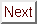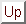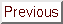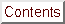Next: Type II/1 Superconductivity Up: Experimental Results: Angular Previous: Extrinsic Influences on

## Type I Superconductivity in C4KHg

The three factors described above, namely sample tilt, mosaic spread, and critical field definition, are the extrinsic influences which may affect the shape of Hc2(theta). None of these is thought to have a large effect on the data. There are also more fundamental, intrinsic explanations for the disparity between data and fit. Probably the most applicable of these to C4KHg is the conceivable existence of type I superconductivity for field orientations near theta = 0°. Type I superconductivity for 0° <= theta <= 25° has been previously reported for C8K by several groups[137,136,141]. C9.4K[137,136] and C8Rb, on the other hand, are found to be type I for all field directions. The existence of type I character for a range of angles in layered superconductors was predicted even before its experimental discovery by Kats. For these reasons the question of type I superconductivity in C4KHg should be approached with an open mind, despite the type II superconductivity reported for all field orientations in Ref. .Figure: Hc2(theta) for C8K, from Ref. . The fields are labeled Hc2 in the type II region and Hc3 and Hc in the type I region.

Iye and Tanuma concluded that there is no type I superconductivity in C4KHg because they did not observe supercooling in their field sweeps for any angle theta. However, the presence of supercooling and the differential paramagnetic effect (DPE) are not the only criteria for the determination of the type of superconductivity. Another approach to the question is to look at the Ginzburg-Landau kappa parameter since kappa = 1/sqrt2 is the critical value dividing type I and type II superconductors. kappa can be estimated using specific heat and critical field data through use of the following formulae:where Lambda is the magnetic field penetration depth, xi is the coherence length, Hc(0) is the zero-temperature thermodynamic critical field, and Gamma is the normal-state linear electronic specific heat coefficient. The equality between kappa and kappa1 is good to within about 20%, so we can use kappa1 as a rough esimate of kappa. (In the discussion below, what is referred to as kappa has actually been calculated using the definition of kappa1.) Because Gamma has been measured for C4KHg, one can estimate kappa(0) by calculating Hc(0) and linearly extrapolating Hc2(T) to zero temperature. The applicability of this procedure to the Tc = 1.5 K GIC's is uncertain because no superconducting transition was observed down to 0.8 K in the samples on which Gamma was measured. It seems possible that Gamma could be different in the Tc = 1.5 K and Tc = 0.7 K samples, even though it is a property of the normal state. Also, Gamma was given in Ref.  in molar units, so in order to convert to cgs units one must calculate the molar volume, which means that one must assume a structure for the compound. Here a (2 × 2)R0° in-plane unit cell is employed, since this is the structure which has most frequently been observed in C4KHg.[123,246] (See Figure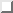.) Despite these caveats, since no other measurement of the specific heat is available, it is useful to see what can be learned by estimating kappa. The results of this calculation are given in Tablefor three MIT C4KHg samples and three from Ref. . Notice that the magnitude of kappa is generally lower for the higher- Tc specimens. The implication is that type I transitions are more likely to be observed in the higher- Tc GIC's.Table: Calculated values of kappa for selected C4KHg samples. The Hc2(0) numbers come from linear extrapolation of the Hc2(T) data (see Section), and so are independent of the Hc2(theta) measurements.

The meaning of kappa will be discussed in more detail below, but for the moment it suffices to say that kappa|| ^c tend to be quite close to kappacritical = 0.707. The primary conclusion from this calculation is that within the uncertainty of the approximations made, the type of the superconductivity in C4KHg is indeterminate, but that it could very well be type I for some samples for theta close to zero. This is especially true at higher temperatures since, according to the two-fluid model:where t is the reduced temperature. Thus it seems quite likely that the presence of type I superconductivity is a factor in explaining the poor fits obtained to Hc2(theta) data with Equation, at least in some specimens at higher temperatures.

Since kappa|| ^c decreases with increasing temperature, the angular range in which type I behavior occurs should increase as t increases. The variation of the angular range of type I character is observed in TaN, the only non-GIC bulk material known to display type I or type II character depending on field orientation. Some Hc2(theta) at constant temperature curves for TaN are shown in Figure. In the bottom trace, at T = 1.65 K, TaN is type II for all angles, as the excellent agreement with the dashed fit indicates. When the temperature is increased to 1.8 K, the sample has type I character near the  direction, with the result that the data there deviate above the dashed curve. When the temperature increase to 2.1 K, the measured critical field for almost all angles theta is Hc, and when the temperature reaches 2.4 K, the sample is type I for all orientations. C8K might also be type I for all orientations for temperatures sufficiently close to Tc. The change with temperature of the Hc2(theta) fits for C4KHg is qualitatively similar, although less dramatic, since type II behavior persists for a much larger range of angles. For the higher- Tc C4KHg specimens, the Hc2(theta) curves are probably most comparable to the 1.8 K TaN trace in Figure.Figure: Hc2(theta) curves for TaN showing a transition from type I to type II character as a function of field direction. From Ref. . TaN is the only bulk superconductor besides C8K (and possibly C4KHg) known to display this variability. The temperatures at which the curves were taken and the thermodynamic critical fields are indicated. Note that at 1.65 K, the sample is entirely type II, but that at 2.4 K it is entirely type I.

If the angular range of type I behavior increases with temperature, then the agreement between Eqn.and the C4KHg data should deteriorate as the temperature increases. The validity of this assertion is demonstrated by Figure, where the fit clearly worsens as the temperature approaches Tc.Figure: Anisotropic Ginzburg-Landau model fits (dotted curves) to Hc2(theta) data as a function of temperature. All fits were produced with the parameters tilt = 0° and mosaic spread = 0°. a) t = 0.29, Hc2,||^c = 47 Oe, anisotropy (1/epsilon) = 9.5, and residual parameter cal R = 1.18. b) t = 0.57, Hc2, ||^c = 33 Oe, anisotropy (1/epsilon) = 5.5, and cal R = 1.25. c) t = 0.78, Hc2,||^c = 23.1 Oe, anisotropy (1/epsilon) = 4.5, and cal R = 1.43.

If type I superconductivity is indeed present in C4KHg, this fact should be accounted for in fitting the data. In Figure, new fits are shown in which the theoretical curve has the form:The idea behind using this functional form is that if Hc is greater than Hc2, then Hc will presumably be measured as the critical field. A quick comparison of Figs.andshows that inclusion of Hc as a parameter dramatically improves the quality of the fits at all temperatures. This large improvement is in contrast to the small (or negative) impact on the quality of fit of the mosaic spread and tilt parameters. Residuals for the fits with and without type I behavior are gathered in Table. The improvement in the residual index is not accounted for simply by the addition of another free parameter since the number of parameters is taken into account in the definition of cal R (see Eqn.).Figure: Anisotropic Ginzburg-Landau model fits (dotted curves) to Hc2(theta) data as a function of temperature, taking into account the possibility of type I behavior. All fits were produced with the parameters tilt = 0° and mosaic spread = 0°. a) t = 0.29, Hc2,||^c = 35 Oe, anisotropy (1/epsilon) = 14, Hc = 65 Oe, and residual parameter cal R = 0.39. b) t = 0.57, Hc2, ||^c = 19 Oe, anisotropy (1/epsilon) = 15.5, Hc = 43 Oe, and cal R = 0.84. c) t = 0.78, Hc2,||^c = 14.5 Oe, anisotropy (1/ epsilon) = 12.5, Hc = 24.5 Oe, and cal R = 1.11.

For these new fits, Hc was taken as a free parameter. The numbers obtained for the thermodynamic critical field from the Hc2(theta) fits for two samples are shown in Table, and Hc(T) is plotted in Figure. According to the BCS theory, Hc(T) should be quadratic, having the form (1 - t2). The fact that the Hc values found from the angular dependence are better fit by a straight line is somewhat disturbing, since (1 - t) behavior is expected for Hc2(T) (see Section). However, Hc(T) showed positive curvature in C8K (see Figure), where signs of type I superconductivity (large hysteresis and differential paramagnetic effect) were unmistakable. Since the temperature dependence of Hc2(T) is unusual in C4KHg, perhaps it is not straining credibility too much to suggest that Hc(T) could be anomalous, too, just as in C8K.

The obvious question is whether these values of the critical field are consistent with those calculated from the specific heat measurements, also given in the table. The calculated thermodynamic critical field tends to be about 1.8 times the fit one. The most likely explanation for this inconsistency is that the value of Gamma quoted in Ref.  is simply too high. For the reasons mentioned above, a higher value of Gamma for the sample of Ref.  would not be very surprising.

Another possible explanation for the difference in magnitude of Hc is that up to this point the demagnetization factor cal D has not been taken into account. (1 - cal D) Hc is the field where normal regions are first formed.[252,157] Thus, the ideal type of magnetization curve shown in Figurea is found only for ideal samples with cal D = 0; in real specimens the sharp corner is rounded. The number, size, and shape of the normal regions formed for (1 - cal D) Hc ;SPMlt; Happlied ;SPMlt; Hc depends typically on sample size, shape, defects, and orientation with respect to the applied field. The primary effect of a non-zero demagnetization factor then is to change the shape of the transition with angle. Because of the method of critical field determination used here (see Figureb), the possible influence of demagnetization on the transition shape cannot be completely ruled out. Therefore, demagnetization-induced distortion could be causing the measured critical field to be substantially less than the actual value of Hc. The influence of demagnetization effects should be particularly strong for the conditions used in the study of C4KHg, since for thin plates in a transverse field (here vecH || ^c) cal D 1.0, meaning that the samples are effectively always in the intermediate state.Table: Hc(t) values for C4KHg obtained from fits to Eqn.. For comparison, Hc(t) calculated from the specific heat data for a Tc = 1.53 K sample is also included. The samples' Hc2(theta) data are shown in Figs.,, and.

Ideally it would be possible to obtain the demagnetization factor as a function of angle in order to correct the Hc2(theta) and Hc(T) curves. Past experimentalists have gotten a value for cal D by machining a material with known critical fields to the size and shape of the sample. Then one gets cal D by comparing the measured critical fields of the machined specimen to the known values for shapes with zero demagnetization. Unfortunately this procedure would have to be carried out for each specimen. If a reliable calculation of cal D were available, it would also allow a more rigorous testing of the theory. However, since the demagnetization is strongly dependent on the sample dimensions and because cal D could easily be affected by such hard-to-quantify factors as exfoliation, the amount of effort required does not seem worthwhile. Any use of a value for the demagnetization would also require the introduction of several new parameters.

An ideal type I superconductor should be easy to distinguish from a type II superconductor because of the discontinuity in its magnetization at <=ft| vecH | = Hc. (See Figure). However, a non-zero demagnetization factor can smear out the magnetization curve, making even type I transitions appear more or less continuous. Despite the presence of demagnetization effects, it seems sensible to examine the field sweeps to see if there is any evidence of discontinuity under the conditions where type I behavior is suspected.

In the lower- Tc C4KHg samples (whose Hc2(theta) curves were well-fit by Eqn.), the experimental traces appear smooth for all angles, as Figurea) shows. The only major difference between theta = 90° and theta = 0° is that the high-angle transitions are considerably broader, for reasons discussed in Section. This result is consistent with the finding that these samples were type II for all orientations, and is consistent with Iye and Tanuma's findings.

In the Tc = 1.5 K samples, the field sweeps for vecH _|_ ^c look smooth, just as they do for the lower- Tc GIC's. For vecH || ^c, on the other hand, the curves appear fairly continuous on the upsweep, but develop a corner near the upper critical field on the downsweep which could be identified as a discontinuity. The arrow in Figureb) indicates the location of the corner. While it is tempting to identify this corner as a discontinuity stemming from a first-order transition, it is a fairly small feature. Therefore, the evidence regarding type I versus type II behavior from the field sweeps is suggestive but ambiguous. Low-temperature magnetization measurements would probably be easier to interpret than susceptibility in this regard, but unfortunately there was no readily available magnetometer that could be cooled to the necessary 1 K range.Figure: Comparison of field sweeps between type II and possible type I transitions. The vertical direction is the inductive voltage, while the horizontal direction is field. All traces taken at about 0.4 K. a) Transitions with vecH _|_ ^c and vecH || ^c for a Tc = 0.95 K sample. For both orientations the transitions appear smooth. b) Transitions with vecH _|_ ^c and vecH || ^c for a Tc = 1.5 K sample. For vecH _|_ ^c, the transition looks smooth, consistent with its expected type II character. For vecH || ^c, on the other hand, there is a small discontinuity in the susceptibility near the upper critical field which is indicated by an arrow. This feature was seen consistently in Tc = 1.5 K samples.Figure: Thermodynamic critical fields obtained from Hc2(theta) fits versus temperature for Tc = 1.5 K C4KHg specimens. The numbers plotted here are the same as in Table. (bigtriangleup), data for a Tc = 1.53 K sample; (circ), data for a Tc = 1.54 K sample; (diamond), a linear fit to the data with Hc(0) = 85.2 g; (circ), a quadratic fit to the data with Hc(0) = 66.5 g; (×), Hc(t) calculated using the specific heat data of Alexander et al., which gives Hc(0) = 112 Oe.

Why should the lower- Tc specimens be type II for all orientations, and the higher- Tc ones be type I for some orientations? The superficial answer is that Hc2 isn't as strongly Tc-dependent as might be expected, since the critical fields of the Tc = 1.5 K samples are only slightly higher than those of the Tc = 0.7 K samples. (Refer to Tablefor the relevant numbers.) If Hc increases as Tc2 and Hc2 increases only weakly with Tc, the net result must be a decrease in kappa with increasing Tc. The deeper answer to the question is that xi and Lambda, the lengths whose ratio determines kappa, are both affected by changes in vF and l, the Fermi velocity and the mean-free-path. Therefore, one can invoke differences between the two sample types either in the Fermi surface or in crystalline perfection to explain dissimilarity of their critical fields. Of course, the same mechanism should ideally also explain their different zero-field transition temperatures. Since the lower- Tc gold specimens have broader zero-field superconducting transitions than the higher- Tc pink samples (see Table), it seems natural to think that the gold samples are less well-ordered. This line of reasoning implies that the gold specimens have a lower mean-free-path. The differences between the gold and pink samples are discussed at length in Chapter. In the meantime, the implications of possible type I superconductivity in C4KHg deserve further consideration.

Another good question about the Hc2(theta) curves is why the data are not perfectly flat near theta = 0 if the measured critical field is Hc there. Some angular dependence of Hc is also present in the TaN data of Figure. As was first suggested by P. Tedrow, the angular dependence of the demagnetization factor could influence the shape of the field sweeps enough to cause a perceived angular dependence of Hc. Koike et al. in their study of C8K also noticed a small angular dependence of Hc near theta = 0 (see Figure). In addition, they measured a strongly angle-dependent supercooling field which they thought must be Hc3, the surface nucleation field. However, Hc3 is not angle-dependent in the sense indicated in Figure; it is in fact equal to 1.69 Hc2(theta), but here theta is the angle between the crystal surface and the c-axis, not the angle between the applied field and the c-axis. Thus Hc3(theta) can only be measured by preparing different crystals with differently oriented surfaces, not by turning the applied magnetic field.

There is another problem with the identification of the angle-dependent supercoooling field with Hc3. The problem is that Hc3 is the surface nucleation field for a field applied parallel to the surface,, while vecH || ^c corresponds to the field perpendicular to the surface. In some anisotropic Chevrel phases with an unusual grain structure, Hc3 has been seen for the ``wrong'' orientation, but for HOPG-based GIC's such as the ones studied by Koike and coworkers, no such microstructural anomalies are anticipated. Therefore the identification of the supercooling field || ^c with Hc3 must be mistaken. It seems likely that the angular dependence of the supercooling field reported in Ref.  is a demagnetization-derived effect, along with the slight angular dependence of Hc observed in C8K and C8Rb. Certainly the change in the demagnetization cal D (from 1 for vecH perpendicular to a plate to 0 for vecH parallel to a plate) is large enough to cause this apparent angular dependence.[242,252]Next: Type II/1 Superconductivity Up: Experimental Results: Angular Previous: Extrinsic Influences on

alchaiken@gmail.com (Alison Chaiken)
Wed Oct 11 22:59:57 PDT 1995